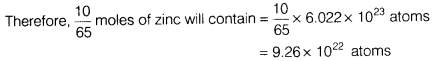# What is meant by atomicity of a molecule?

• What is meant by atomicity of a molecule? Give one example of triatomic molecule.
• Sodium carbonate (Na2CO3 . 10H2O) is an important industrial chemical. Calculate its atomic mass. [Atomic mass of Na = 23 u, C = 12 u, O = 16u and H = 1 u]
1. What is the number of zinc (Zn) atoms in a piece of zinc weighing 10 g?
• Atomicity of a molecule is defined as the number of atoms present in a molecule. e.g. Ozone (O3) is a triatomic molecule.
• Atomic mass of sodium carbonate (Na2CO3 . 10H2O) = 2Na + 1C + 3O + 10(2H + 10)
= 2 x 23 + 12 + 3 x 16 + 10 (2 x 1 + 16)
= 46 + 12 + 48 + 180 = 286 u
1. Number of moles of zinc (Zn)1 mole of zinc contains 6022 x 1023 atoms